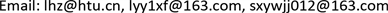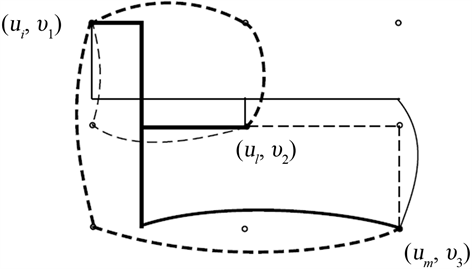﻿ 完全图的笛卡尔积的广义3-连通度 The Generalized 3-Connectivity of Cartesian Product of Complete Graphs

Advances in Applied Mathematics
Vol. 08  No. 02 ( 2019 ), Article ID: 29025 , 7 pages
10.12677/AAM.2019.82036

The Generalized 3-Connectivity of Cartesian Product of Complete Graphs

Hengzhe Li, Yuanyuan Lu, Jiajia Wang

College of Mathematics and Information Science, Henan Normal University, Xinxiang HenanReceived: Feb. 3rd, 2019; accepted: Feb. 18th, 2019; published: Feb. 26th, 2019ABSTRACT

Let S be a set of at least two vertices in a graph G. A subtree T of G is an S-Steiner tree if $S\subseteq V\left(T\right)$ . Two S-Steiner trees ${T}_{1}$ and ${T}_{2}$ are internally disjoint if $E\left({T}_{1}\right)\cap E\left({T}_{2}\right)=\varnothing$ and $V\left({T}_{1}\right)\cap V\left({T}_{2}\right)=S$ . Let ${\kappa }_{G}\left(S\right)$ be the maximum number of internally disjoint S-Steiner trees in G, and let ${\kappa }_{k}\left(G\right)$ be the minimum ${\kappa }_{G}\left(S\right)$ for S ranges over all k-subsets of $V\left(G\right)$ . In this paper, we study the ${\kappa }_{3}$ -connectivity of Cartesian product of complete graphs, determine ${\kappa }_{3}\left({K}_{{n}_{1}}\square {K}_{{n}_{2}}\right)={n}_{1}+{n}_{2}-3$ for any two complete graphs; ${\kappa }_{3}\left({K}_{{n}_{1}}\square {K}_{{n}_{2}}\square \cdots \square {K}_{{n}_{k}}\right)={\sum }_{i=1}^{k}{n}_{i}-k-1$ for any k complete graphs, where $k\ge 2$ .

Keywords:Complete Graphs, ${\kappa }_{3}$ -Connectivity, Cartesian ProductCopyright © 2019 by authors and Hans Publishers Inc.1. 引言

2. 预备知识

3. 主要定理及其证明

$x=\left({u}_{i},{v}_{1}\right),y=\left({u}_{l},{v}_{2}\right),z=\left({u}_{m},{v}_{3}\right)$${S}^{\prime }=\left\{\left({u}_{c},{v}_{j}\right)|c=i,l,m;j=1,2,3\right\}$ 。考虑如下两种情况。Figure 1. Solid lines in bold for ${T}_{j}$ , $1\le j\le a$ ; Solid lines for ${{T}^{\prime }}_{j}$ , $1\le j\le {n}_{k}-3$ ; Dashed lines for ${{T}^{\prime }}_{{n}_{k}-2}$ ; Dashed lines in bold for ${{T}^{\prime }}_{{n}_{k}-1}$

The Generalized 3-Connectivity of Cartesian Product of Complete Graphs[J]. 应用数学进展, 2019, 08(02): 320-326. https://doi.org/10.12677/AAM.2019.82036

1. 1. Bondy, J.A. and Murty, U.S.R. (2008) Graph Theory, GTM 244. Springer, Berlin.

2. 2. Chartrand, G., Kapoor, S.F., Lesniak, L. and Lick, D.R. (1984) Generalized Connectivity in Graphs. Bulletin of the Bombay Mathematical Colloquium, 2, 1-6.

3. 3. Chiue, W.-S. and Shieh, B.-S. (1999) On Connectivity of Cartesian Product of Two Graphs. Applied Mathematics and Computation, 102, 129-137. https://doi.org/10.1016/S0096-3003(98)10041-3

4. 4. Imrich, W. and Klavžar, S. (2000) Product Graphs. Structure and Recognition. Wiley-Interscience Series in Discrete Mathematics and Optimization, Wiley, New York.

5. 5. Imrich, W., Klavžar, S. and Rall, D.F. (2008) Topics in Graph Theory. Graphs and Their Cartesian Product. AK Peters, Wellesley.

6. 6. Klavžar, S. and Spacapan, S. (2008) On the Edge-Connectivity of Cartesian Product Graphs. Asian-European Journal of Mathematics, 1, 93-98.

7. 7. Sabidussi, G. (1957) Graphs with Given Group and Given Graph-Theoretic Properties. Canadian Journal of Mathematics, 9, 515-525. https://doi.org/10.4153/CJM-1957-060-7

8. 8. Špacapan, S. (2008) Connectivity of Cartesian Products of Graphs. Applied Mathematics Letters, 21, 682-685. https://doi.org/10.1016/j.aml.2007.06.010

9. 9. Xu, J. and Yang, C. (2006) Connectivity of Cartesian Product Graphs. Discrete Mathematics, 306, 159-165. https://doi.org/10.1016/j.disc.2005.11.010

10. 10. Chartrand, G., Okamoto, F. and Zhang, P. (2010) Rainbow Trees in Graphs and Generalized Connectivity. Networks, 55, 360-367.

11. 11. Li, H. and Wang, J. (2018) The λ3-Connectivity and κ3-Connectivity of Recursive Circulants. Applied Mathematics and Computation, 339, 750-757. https://doi.org/10.1016/j.amc.2018.07.065

12. 12. Li, H., Li, X. and Sun, Y. (2012) The Generalied 3-Connectivity of Cartesian Product Graphs. Discrete Mathematics and Theoretical Computer Science, 14, 43-54.

13. 13. Li, S., Li, X. and Zhou, W. (2010) Sharp Bounds for the Generalized Connectivity κ3(G). Discrete Mathematics, 310, 2147-2163. https://doi.org/10.1016/j.disc.2010.04.011

14. 14. Li, X. and Mao, Y. (2014) The Generalized 3-Connectivity of Lexicographic Product Graphs. Discrete Mathematics and Theoretical Computer Science, 16, 339-354.

15. 15. Li, X., Mao, Y. and Sun, Y. (2014) On the Generalized (Edge-)Connectivity of Graphs. Australasian Journal of Combinatorics, 58, 304-319.

16. 16. Li, H., Wu, B., Meng, J. and Ma, Y. (2018) Steiner Tree Packing Number and Tree Connectivity. Discrete Mathematics, 341, 1945-1951. https://doi.org/10.1016/j.disc.2018.03.021

17. 17. Li, H., Ma, Y., Yang, W. and Wang, Y. (2017) The Generalized 3-Connectivity of Graph Products. Applied Mathematics and Computation, 295, 77-83. https://doi.org/10.1016/j.amc.2016.10.002

18. 18. Li, X. and Mao, Y. (2016) Generalized Connectivity of Graphs. In: Springer Briefs in Math, Springer, New York. https://doi.org/10.1007/978-3-319-33828-6

19. 19. Dirac, G.A. (1960) In abstrakten graphen vorhandene voll-standige 4-graphen und ihre unterteilungen. Mathematische Nachrichten, 22, 61-85. https://doi.org/10.1002/mana.19600220107

20. 20. Lin, S. and Zhang, Q. (2017) The Generalized 4-Connectivity of Hypercubes. Discrete Applied Mathematics, 220, 60-67. https://doi.org/10.1016/j.dam.2016.12.003